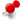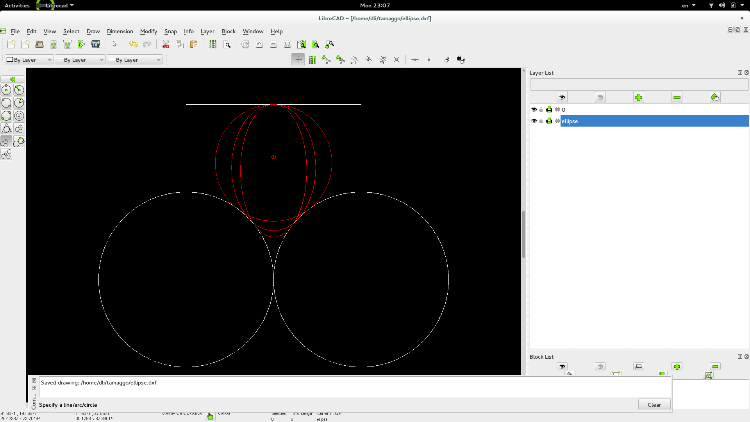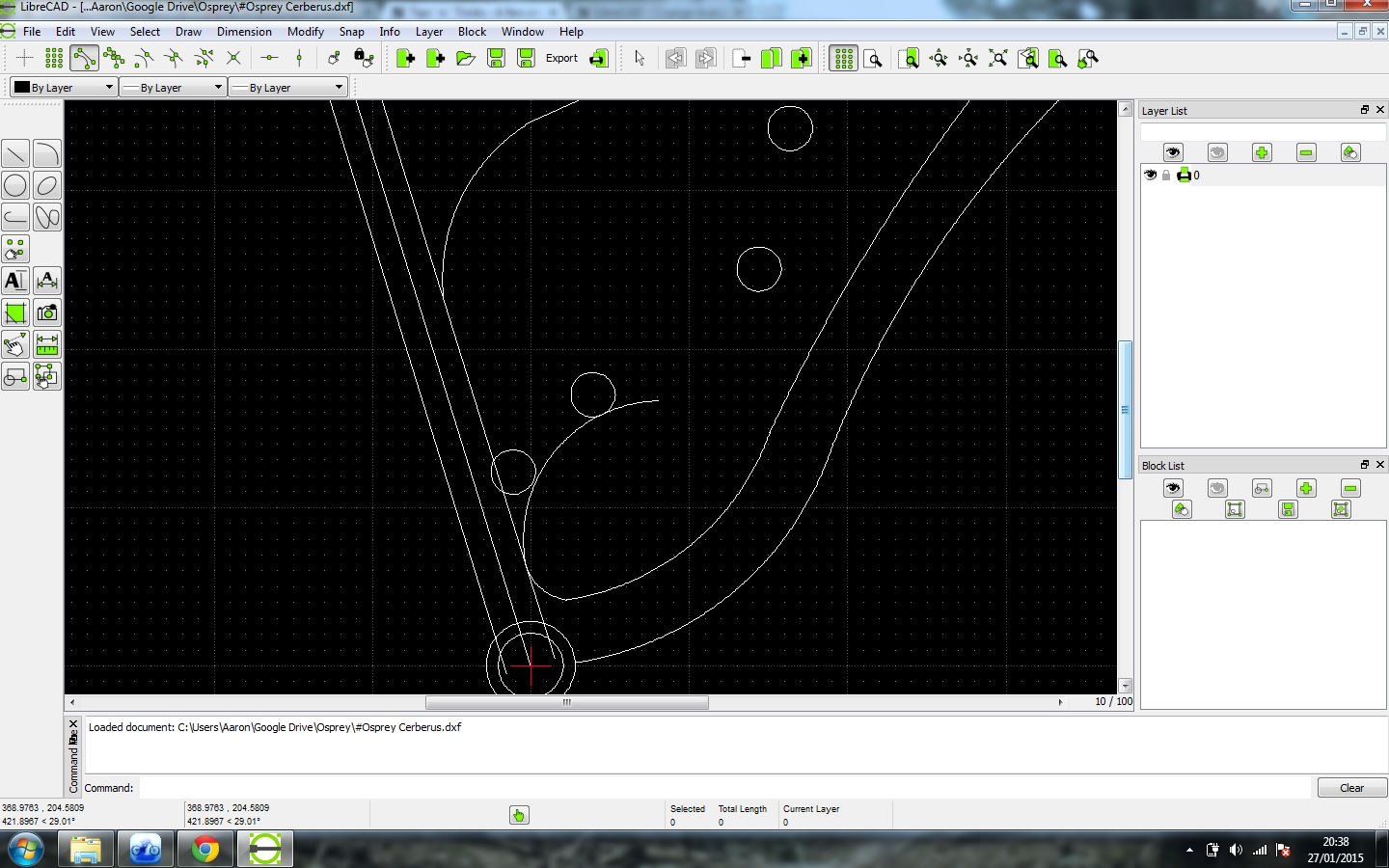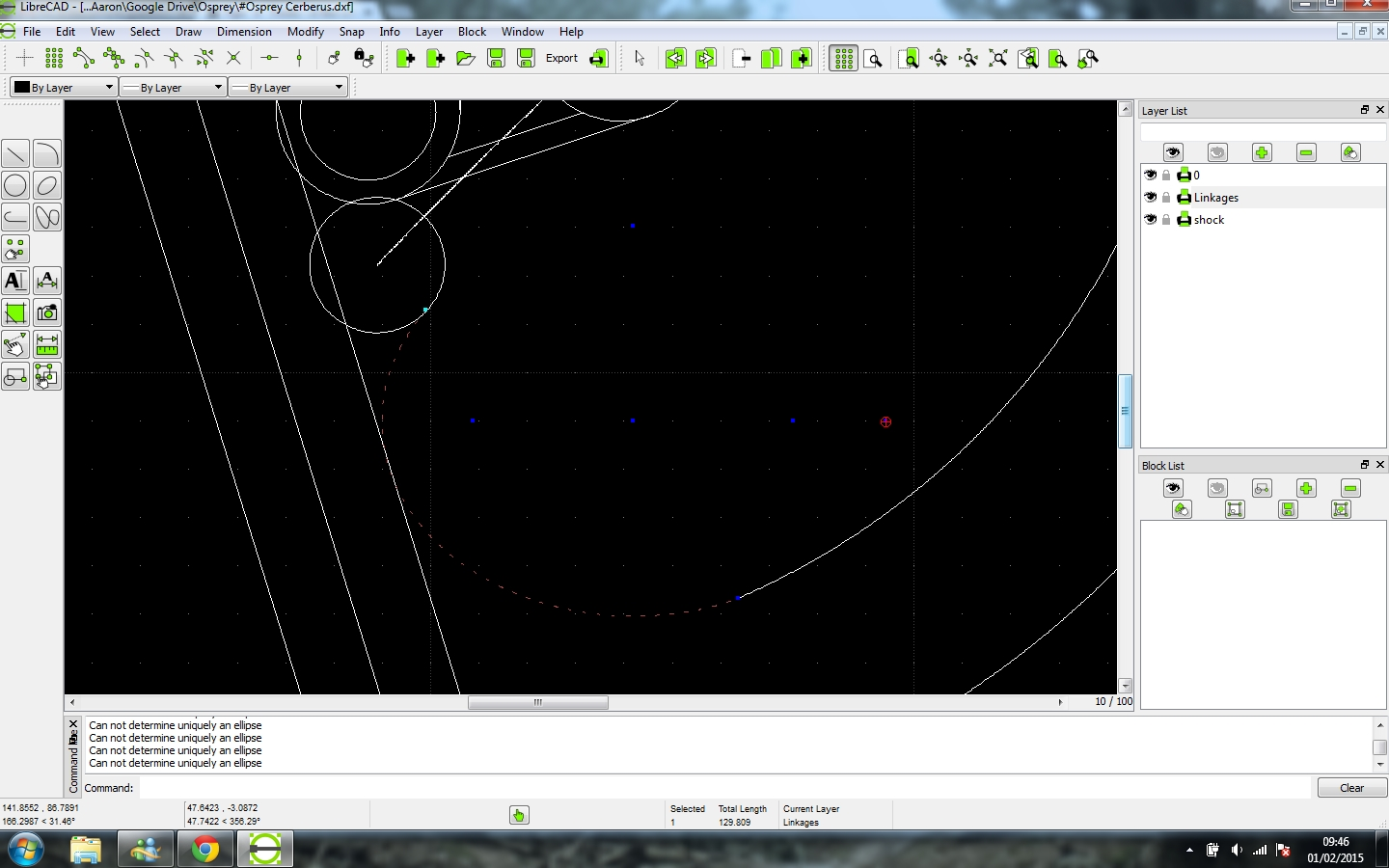# A few simple questionsClassicListThreaded9 messagesOpen this post in threaded view
|

## A few simple questions

 Hi all, I've just started putting together a design and I've a few questions which should be pretty easy to answer. How do I trim a circle to create the ends points as the tangents with other circles? Can I create an ellipse that is tangential to two circles and a line? Thanks. OLC
Open this post in threaded view
|

## Re: A few simple questions

 to trim by tangential points could be tricky. It may help to draw a construction line (say, by linking the centers of the two tangential circles), and trim by line. Second question: "create an ellipse that is tangential to two circles and a line"? To me, this is not a simple question at all. Given 2 circles and a line, there could be as many as 4 possible common tangential circles (which are tangential to the 2 given circle and the given line). Now, consider a tangential ellipse, instead of tangential circles, there are two more degree of freedom (say, the eccentricity and the orientation). Therefore, there are always infinite number of tangential ellipses, if there's existence of one tangential circle.oneloosecrank wrote Hi all, I've just started putting together a design and I've a few questions which should be pretty easy to answer. How do I trim a circle to create the ends points as the tangents with other circles? Can I create an ellipse that is tangential to two circles and a line? Thanks. OLC
Open this post in threaded view
|

## Re: A few simple questions

 Ah, well - here is the curve I'm trying to achieve:So I have an arc attempting to connect the two circles and the 73deg line, and I'd like to be able to adjust the oval's orientation to get the desired curve - then trim down the curve so that it ends at the tangent point against the middle most circle. Any advice on getting this curve to meet the straight line and the two circles? Thanks
Open this post in threaded view
|

## Re: A few simple questions

 You can draw a tangential circle given (2 circles + 1 line). Try main menu: Draw -> Circle -> Tangential 3 Circles it says "3 Circles", in the sense of generalized circles: http://en.wikipedia.org/wiki/Generalised_circleAt each step, you can select either a line or a circle. This "3 Circles" could be buggy due to limited robustness of our equation solver, but we can keep improving it. oneloosecrank wrote Ah, well - here is the curve I'm trying to achieve:So I have an arc attempting to connect the two circles and the 73deg line, and I'd like to be able to adjust the oval's orientation to get the desired curve - then trim down the curve so that it ends at the tangent point against the middle most circle. Any advice on getting this curve to meet the straight line and the two circles? Thanks
Open this post in threaded view
|

## Re: A few simple questions

 Excellent, I've gotten the ellipse I need connecting the line and curves, thanks :)On 27 January 2015 at 21:35, dxli [via LibreCAD] wrote: You can draw a tangential circle given (2 circles + 1 line). Try main menu: Draw -> Circle -> Tangential 3 Circles it says "3 Circles", in the sense of generalized circles: http://en.wikipedia.org/wiki/Generalised_circleAt each step, you can select either a line or a circle. This "3 Circles" could be buggy due to limited robustness of our equation solver, but we can keep improve it. oneloosecrank wrote Ah, well - here is the curve I'm trying to achieve:So I have an arc attempting to connect the two circles and the 73deg line, and I'd like to be able to adjust the oval's orientation to get the desired curve - then trim down the curve so that it ends at the tangent point against the middle most circle. Any advice on getting this curve to meet the straight line and the two circles? Thanks If you reply to this email, your message will be added to the discussion below: http://forum.librecad.org/A-few-simple-questions-tp5711021p5711032.html To unsubscribe from A few simple questions, click here. NAML
Open this post in threaded view
|

## Re: A few simple questions

 One other question, I can't parallel line an ellipse - but it will parallel line a curve and a circle. Any thoughts on what I've missed?
Open this post in threaded view
|

## Re: A few simple questions

 In reply to this post by oneloosecrank I just fixed some failure cases in "Draw tangential 3", and you should be able to draw a tangential circle, given (two circles, 1 line). Could you explain how you got a tangential ellipse for (two circles, one line)? oneloosecrank wrote Excellent, I've gotten the ellipse I need connecting the line and curves, thanks :)Through cheating unfortunately. hopefully the image shows clearly where I've place my points having used the end of line and entity snaps, then I've just stretched the ellipse until it is effectively tangent to the line. It would be nice If I could have the edge of the ellipse snap to the line but it's accurate enough for my needs. Libre cad is reporting that it cannot 'uniquely determine an ellipse', or part of an ellipse to allow me to create a parallel line. Is this expected behaviour?